# Problems of the Week

Contribute a problem

You attempt a trick shot by striking the cue ball below its center of mass, aiming away from the striped ball. Your hope is to give the cue ball enough backspin so that it will return and knock the striped ball into the corner pocket.

How can you guarantee that the cue ball will always return to hit the striped ball into the corner?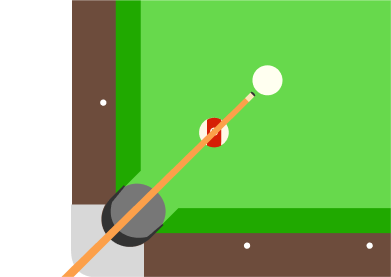Details and Assumptions:

• The cue ball has mass $m$ and radius $r,$ the initial velocity you give it is $v_0,$ and the backspin you give it is $\omega_0.$
• Treat the cue ball as a perfect solid sphere.
• The collision between the cue stick and cue ball is perfectly elastic.
• You hit the ball such that it is always in contact with the ground.

A fox moving at a constant speed of $s$$\text{ m/s}$ wants to eat a duck that is swimming at $1 \text{ m/s}$ in a pond. The duck can only fly when he’s on land, and the fox can’t swim.

What is the maximal value of $s$ such that wherever the animals start, the duck can reach land without being caught by the fox?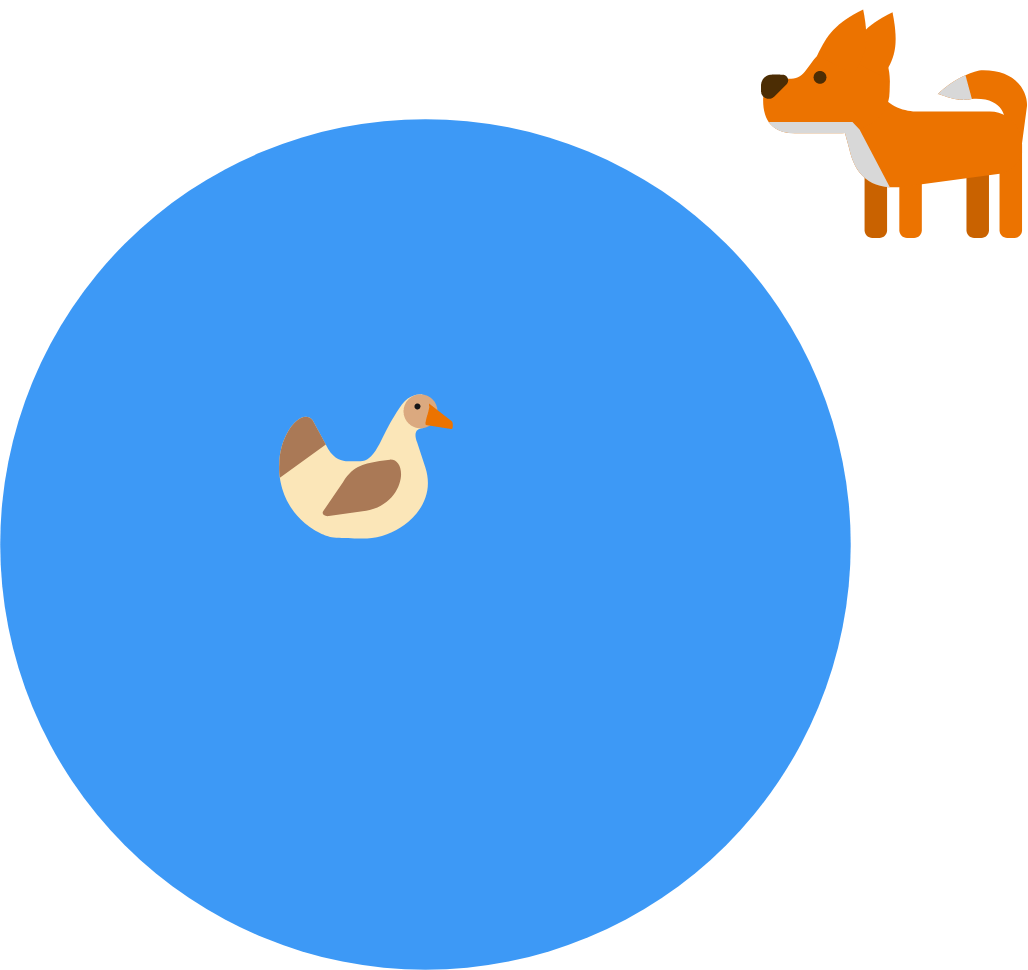Assumptions:

• The pond is a perfectly round circle.
• The fox can start anywhere except inside the pond, but he can never enter the pond.
• The duck must start somewhere on the pond.
• If the duck reaches the edge of the pond at the exact same time as the fox, the fox catches the duck.
• If the duck reaches land and the fox is not there, he instantly flies away and the fox loses his meal.
• The duck and the fox see each other and take decisions in real time.

Four distinct positive integers $a$, $b$, $c$, and $d$ are such that $a+b+c+d=1000.$ Find the minimum value of $\text{lcm}(a,b,c,d).$

A $4\times 4\times 4$ grid has just enough material removed such that the front side projects an $\text{X},$ the right side a $\text{Y},$ and the top side a $\text{Z}.$ The orthogonal views of these letters are shown below.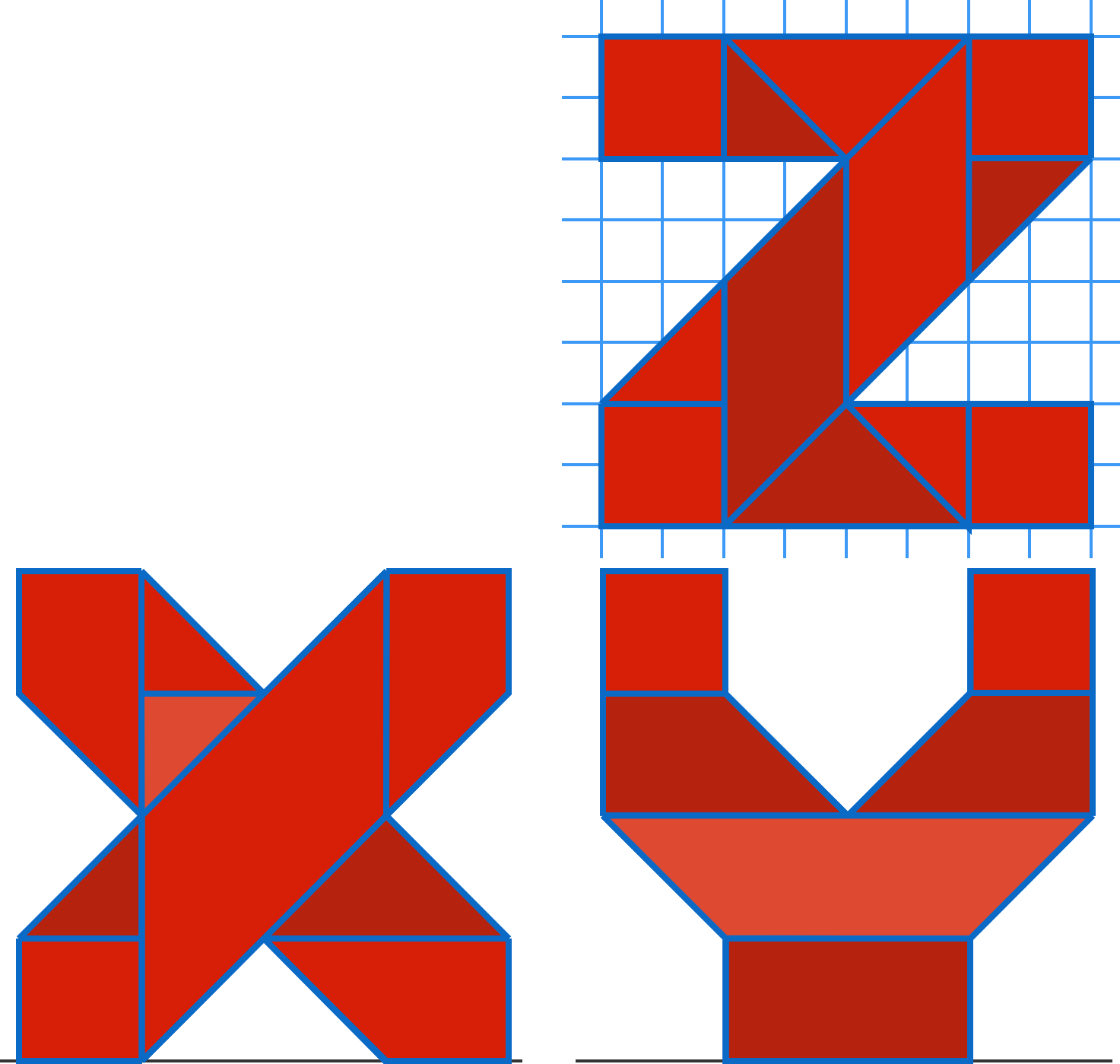An isometric view of the final shape is shown below.

What is the volume of this figure?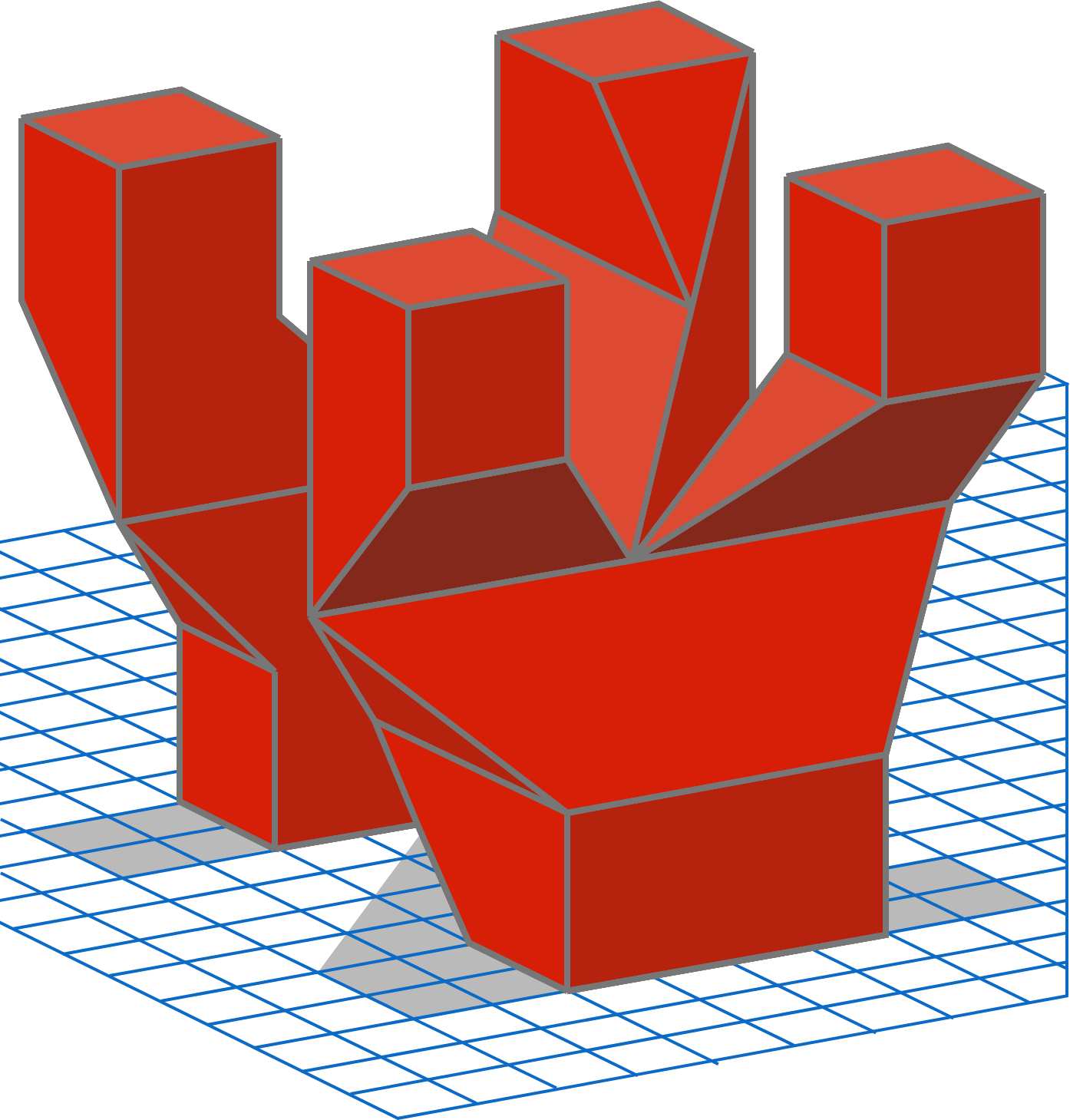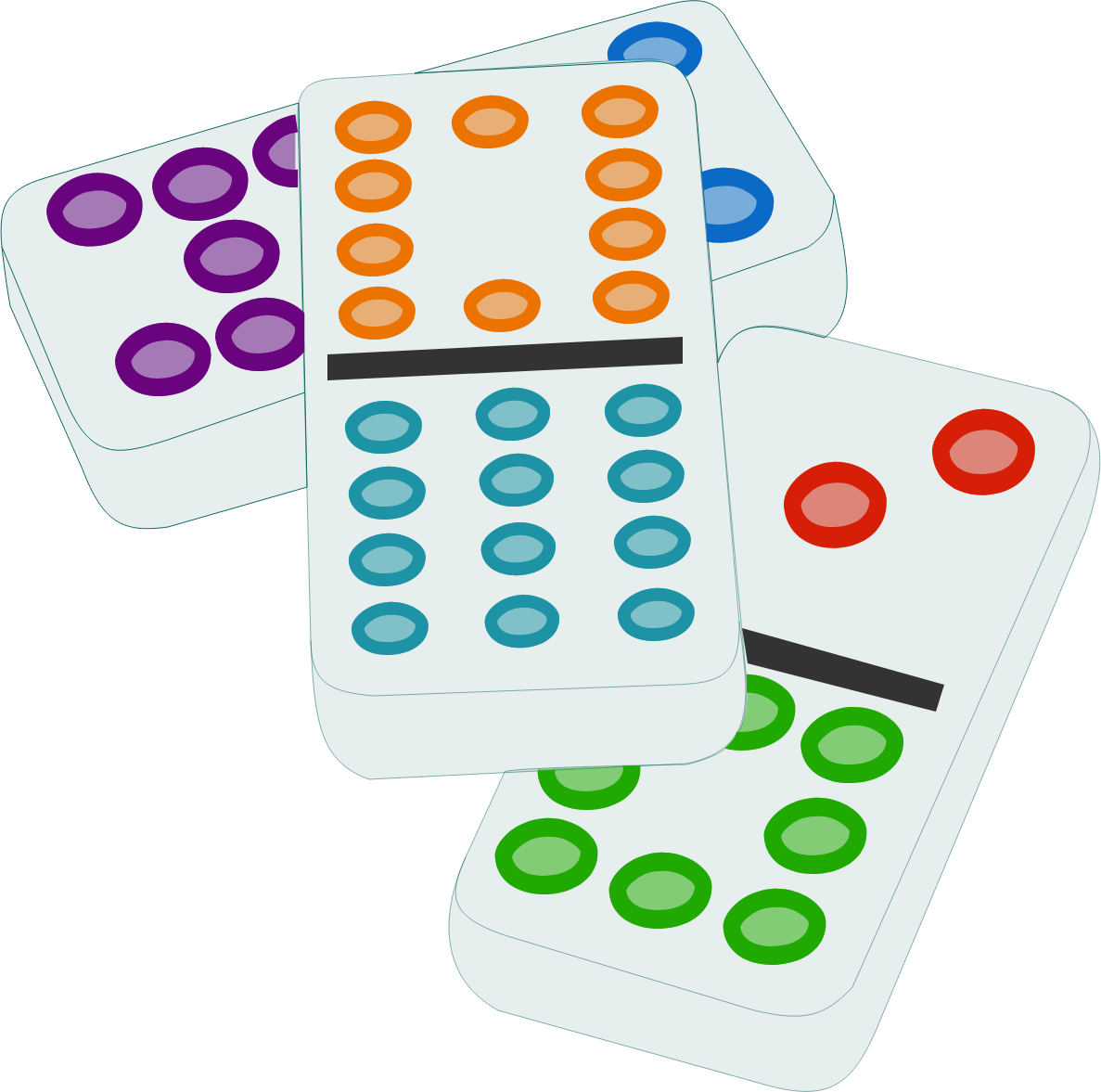A single domino is a rectangular tile divided into two square ends marked with spots. A domino set of order $n$ consists of all the domino tile combinations with spot counts from $0$ to $n$ inclusive.

$T(n)$ is the sum of the totals of the two spot counts on each tile in the domino set of order $n$, and $P(n)$ is the sum of the products of the two spot counts on each tile in the domino set of order $n$.

For example, a domino set of order $2$ would consist of the domino tiles with numbers $(0, 0)$, $(0, 1)$, $(0, 2)$, $(1, 1)$, $(1, 2)$, $(2, 2)$, such that \begin{aligned} T(2) &= (0 + 0) + (0 + 1) + (0 + 2) + (1 + 1) + (1 + 2) + (2 + 2) \\&= 12\\\\ P(2) &= (0 \cdot 0) + (0 \cdot 1) + (0 \cdot 2) + (1 \cdot 1) + (1 \cdot 2) + (2 \cdot 2) \\&= 7. \end{aligned} What is $\lim_{n\to\infty}} \frac{n T(n)}{P(n)}$

×